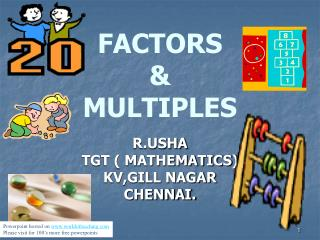# FACTORS & MULTIPLES - PowerPoint PPT PresentationDownload PresentationFACTORS & MULTIPLES

FACTORS & MULTIPLES
Download Presentation## FACTORS & MULTIPLES

- - - - - - - - - - - - - - - - - - - - - - - - - - - E N D - - - - - - - - - - - - - - - - - - - - - - - - - - -
##### Presentation Transcript

1. FACTORS & MULTIPLES R.USHA TGT ( MATHEMATICS) KV,GILL NAGAR CHENNAI. Powerpoint hosted on www.worldofteaching.com Please visit for 100’s more free powerpoints

2. ARRANGEMENT OF STARS Take 6 stars and arrange them in different ways

3. 1 Row × 6 Stars/Row = 6 Stars

4. 3 Rows × 2 Stars per row = 6 stars

5. 2 Rows × 3 stars per row = 6 stars

6. 6 Rows × 1 Star/Row = 6 Stars

7. As we can see, 6 can be written as product of two numbers in many ways: 6 = 1 × 6 6 = 2 × 3 6 = 3 × 2 6 = 6 × 1 1, 2, 3 and 6 are exact divisors of 6

8. ARRANGEMENT OF FOOTBALLS Take 8 footballs and arrange them in different ways

9. Arrangement of 8 in different ways 1 Row × 8 Balls/Row = 8 Balls

10. 2 Rows × 4 Balls / Row = 8 Balls

11. 4 Rows × 2 Balls / Row = 8 Balls

12. 8 Rows × 1 Ball / Row = 8 Balls

13. 1, 2, 4 and 8 are exact divisors of 8.

14. FACTORS OF 28 28 ÷ 1 = 28 28 ÷ 4 = 7 28 ÷ 7 = 4 28 ÷ 28= 1 1 4 7 28

15. FACTORS OF 16 16 ÷ 1 = 16 16 ÷ 2 = 8 16 ÷ 4 = 4 16 ÷ 8 = 2 16 ÷ 16 = 1 1 2 4 8 16

16. FACTOR A factorof a number is an exact divisor of that number i.e. the factor leaves remainder 0 when it divides the number.

17. 4 × 5 = 20 4 and 5 are factors of 20. We can also say that 20 is multiple of 4 and 5. In 2 × 12 = 24, 2 and 12 are factors of 24. 24 is the multiple of 2 and 12. So, a number is a multiple of its factors.

18. Multiples of 2 = 2,4,6,8,10,12,14,16,18,20,22,24… Multiples of 3 = 3,6,9,12,15,18,21,24,27,30,33,36,… Multiples of 15 = 15,30,4,60,75,90,105,120,135,…… The number of multiples of a given number is infinite.

19. FACTORS OF SOME NUMBERS 8 = 1,2,4,8 16 = 1,2,4,8,16 25 = 1,5,25

20. 4 × 5 = 20 Factors Multiple

21. POINTS TO REMEMBER • 1 is a factor of every number. • Every factor of a number is an exact divisor of that number. • Every factor is less than or equal to the given number. • Number of factors of a given number are finite.

22. POINTS TO REMEMBER contd • Every multiple of a number is greater than or equal to that number. • The number of multiples of a given number is infinite. • Every number is a multiple of itself.

23. FACTOR OR MULTIPLE? For each of numbers in the statements, select either multiple [M] or factor [F] • 34 × 5 = 170 [F] [M] [F] [M] [F] [M] • 25 × 4 = 100 [F] [M] [F] [M] [F] [M] • 90 × 10 = 900 [F] [M] [F] [M] [F] [M] • 45 × 6 = 270 [F] [M] [F] [M] [F] [M] • 7 × 30 = 210 [F] [M] [F] [M] [F] [M]

24. State whether the following statements are TRUE or FALSE 1. 1 is a factor of every number. FT 2. The largest factor of any number is the number itself. FT 3. Number of factors of a given number is infinite. FT 4.The number of multiples of 5 is 10. FT 5.The factors of 65 are 1, 5 and 13 only. FT ( Click ‘F’ for false and ‘T’ for true )

25. THANK YOU

26. CORRECT

27. WRONG